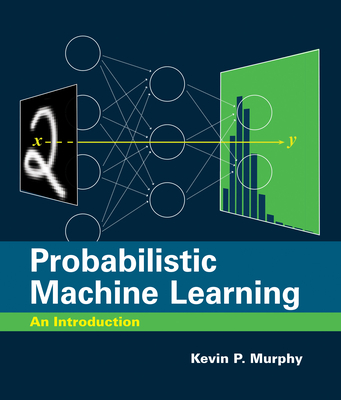### Probabilistic Machine Learning: An Introduction### Huge savings for students

Each student receives a 50% discount off of most books in the HSG Book Store. During class, please ask the instructor about purchase details.
 List Price: \$110.00 Price: \$55.00 You Save: \$55.00
7A detailed and up-to-date introduction to machine learning, presented through the unifying lens of probabilistic modeling and Bayesian decision theory.

This book offers a detailed and up-to-date introduction to machine learning (including deep learning) through the unifying lens of probabilistic modeling and Bayesian decision theory. The book covers mathematical background (including linear algebra and optimization), basic supervised learning (including linear and logistic regression and deep neural networks), as well as more advanced topics (including transfer learning and unsupervised learning). End-of-chapter exercises allow students to apply what they have learned, and an appendix covers notation.

Probabilistic Machine Learning grew out of the author's 2012 book, Machine Learning: A Probabilistic Perspective. More than just a simple update, this is a completely new book that reflects the dramatic developments in the field since 2012, most notably deep learning. In addition, the new book is accompanied by online Python code, using libraries such as scikit-learn, JAX, PyTorch, and Tensorflow, which can be used to reproduce nearly all the figures; this code can be run inside a web browser using cloud-based notebooks, and provides a practical complement to the theoretical topics discussed in the book. This introductory text will be followed by a sequel that covers more advanced topics, taking the same probabilistic approach.

MIT Press